### Home > CALC > Chapter 1 > Lesson 1.2.1 > Problem1-27

1-27.
1. Sketch the graph of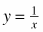. 1-27 HW eTool (Desmos). Homework Help ✎

1. Why does this graph have a vertical asymptote? What is the equation of that asymptote?

2. State the equation of the horizontal asymptote.

3. Alterso that the vertical asymptote is x = 1 and the horizontal asymptote is y = 3.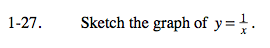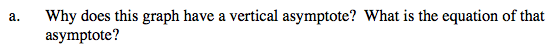Vertical asymptotes are lines with undefined slopes. Their equations are of the form: x = k where k is a constant.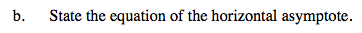Consider the horizontal lines that you found in problem 1-22(b).

Horizontal asymptotes are lines with 0 slope. Their equations are of the form: y = 0x+b or y = k, where k is some constant.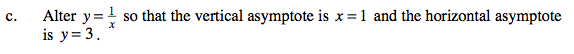$y=\frac{1}{x-h}+k$

Use the eTool below to view the graphs.
Click the link at right for the full version of the eTool: Calc 1-27 HW eTool